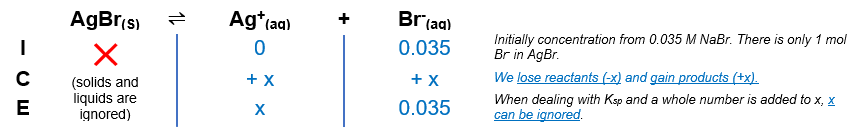# Problem: Calculate the molar solubility of AgBr in 0.035 M NaBr (Ksp = 5 × 10–13).

###### FREE Expert Solution

Notice that there is a common ion present, Br-. We can construct an ICE table for the dissociation of AgBr. Remember that solids are ignored in the ICE table.The Ksp expression for AgBr is:

$\overline{){{\mathbf{K}}}_{{\mathbf{sp}}}{\mathbf{=}}\frac{\mathbf{products}}{\overline{)\mathbf{reactants}}}{\mathbf{=}}\mathbf{\left[}{\mathbf{Ag}}^{\mathbf{+}}\mathbf{\right]}\mathbf{\left[}{\mathbf{Br}}^{\mathbf{-}}\mathbf{\right]}}$

Note that each concentration is raised by the stoichiometric coefficient: both [Ag+] and [Br] are raised to 1.

85% (128 ratings)###### Problem Details

Calculate the molar solubility of AgBr in 0.035 M NaBr (Ksp = 5 × 10–13).

Frequently Asked Questions

What scientific concept do you need to know in order to solve this problem?

Our tutors have indicated that to solve this problem you will need to apply the Ksp concept. You can view video lessons to learn Ksp. Or if you need more Ksp practice, you can also practice Ksp practice problems.

What professor is this problem relevant for?

Based on our data, we think this problem is relevant for Professor Staff's class at NIU.

What textbook is this problem found in?

Our data indicates that this problem or a close variation was asked in Chemistry - OpenStax 2015th Edition. You can also practice Chemistry - OpenStax 2015th Edition practice problems.